Get instant live expert help with Excel or Google Sheets“My Excelchat expert helped me in less than 20 minutes, saving me what would have been 5 hours of work!”

#### Post your problem and you’ll get expert help in seconds.

Your message must be at least 40 characters
Our professional experts are available now. Your privacy is guaranteed.

# Top Reasons Why Your VLOOKUP is Not Working, and How to Correct Them

VLOOKUP is very common, popular and widely used function in Excel and Google Sheets. But the majority of users complain that VLOOKUP is not working correctly or giving incorrect results. This is because of some limitations with the VLOOKUP function, and sometimes users also do not carefully follow its rules and syntax. Here, we are going to discuss some of the common errors and reasons why VLOOKUP does not work.

## Common VLOOKUP Errors

In this article, we are going to discuss VLOOKUP errors, like #NA, #VALUE, #REF, and VLOOKUP returning incorrect results. Now you are going to see the reasons for these errors and their solutions.

### VLOOKUP #NA error

When VLOOKUP formula cannot find a match, then this error displays, meaning “not available.” But it is always not correct that the lookup value is actually not available. There could be some reasons why VLOOKUP returns this error.

• Extra Spaces in Lookup Value. This is one of the most common reasons behind the #NA error in VLOOKUP. In big data set it is very hard to identify these leading or trailing spaces in lookup values that cause the VLOOKUP function to not find the match and return #NA error.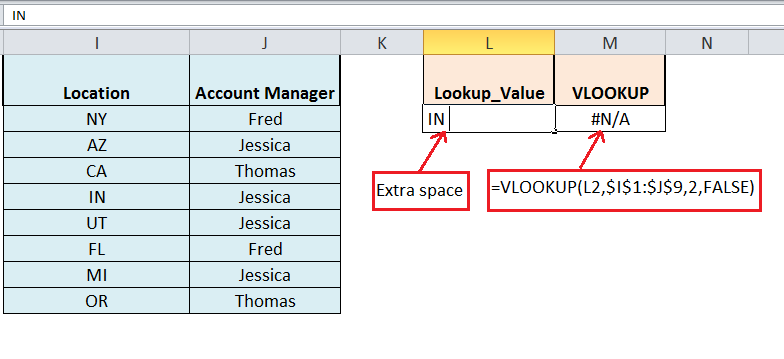Solution:

To kill these extra spaces, you need to wrap the Lookup_value argument in the VLOOKUP formula with the TRIM function to ensure correct working of the function, such as;

`=VLOOKUP(TRIM(L2),\$I\$1:\$J\$9,2,FALSE)`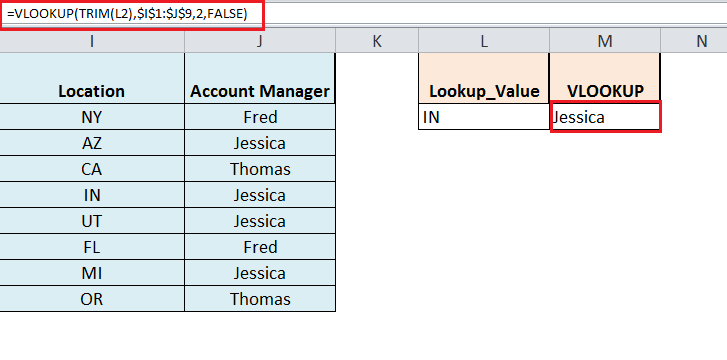• Typo mistake in Lookup_Value. If you wrongly enter the value in the lookup_value argument of VLOOKUP function, then it generates the #NA error. So you must enter the lookup value correctly in the lookup_value argument.
• Numeric values are formatted as Text. If numeric values are formatted as text in a table_array argument of VLOOKUP function, then it comes up with the #NA error.

To fix this error, you must check and properly format the numeric values as “Number.”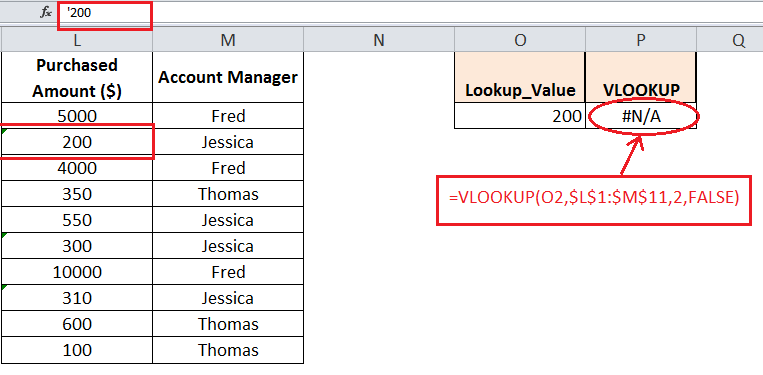• Lookup Value not in First column of table array. As per rule lookup value must be in the first (leftmost) column of a table_array argument of the VLOOKUP function. If lookup value is not present in the first column of a table_array, then VLOOKUP generates #NA error.

To fix this error, you must arrange your columns correctly and then select your table_array in VLOOKUP function.

• In case of Approximate Match typeIn case of approximate match type (TRUE), your VLOOKUP function generates #NA error if your lookup value is smaller than the smallest value available in the first column of table_array.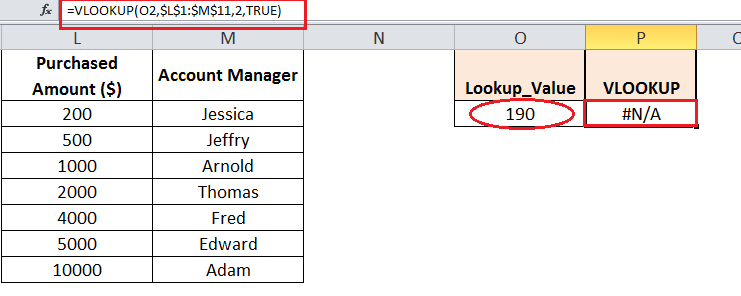### VLOOKUP #VALUE error

Generally, if you enter wrong data type in the formula in Excel, then formula generates #Value error. But in the case of VLOOKUP function, there are following three reasons that should look into.

• Index_number  less than 1. If you enter index_number  argument less than 1 in VLOOKUP function, then it returns a #VALUE error. So you must check index_number argument if VLOOKUP argument returns this error.
• Workbook path is incorrect or incomplete: When you supply the table_array from another workbook in VLOOKUP and path of that workbook is incomplete then VLOOKUP returns a #VALUE error. So you need to follow its following syntax to provide it fully.

`=VLOOKUP(lookup_value, '[workbook name]sheet name'!table_array, col_index_num, FALSE)`

If anything in the path format is missing, VLOOKUP formula returns a #VALUE error, unless the lookup workbook is currently open.

• Lookup value characters length. VLOOKUP supports a maximum of 255 characters length of a lookup value argument. If lookup value character length exceeds this limit in VLOOKUP, then formula returns a #VALUE error.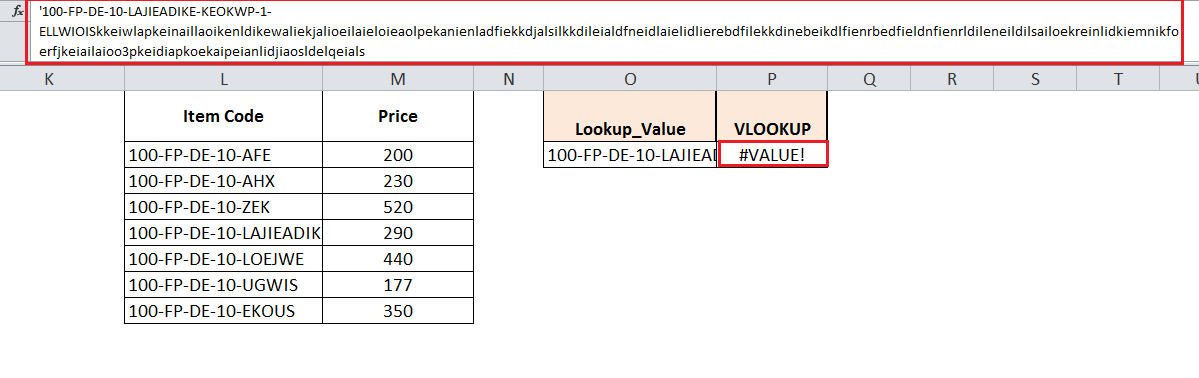Solution:

Either you can reduce the character length of the lookup value to the maximum limit of 255 characters in the VLOOKUP function or you should use INDEX, MATCH formula instead of the VLOOKUP function in the following pattern;

`=INDEX (returing_range,MATCH(TRUE,INDEX(lookup_range = lookup_value,0),0))`

`=INDEX(\$M\$2:\$M\$8,MATCH(TRUE,INDEX(\$L\$2:\$L\$8=O2,0),0))`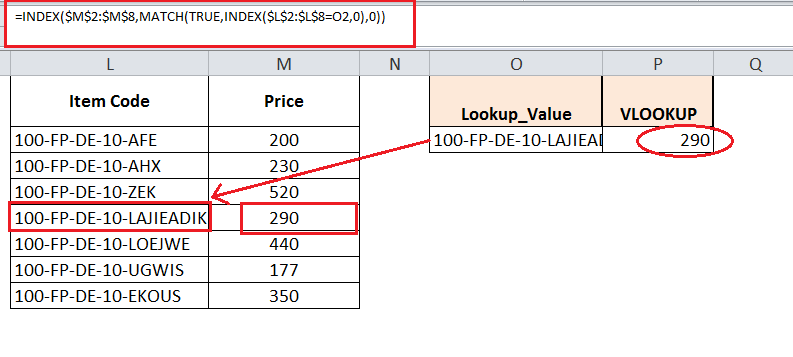### VLOOKUP #REF error

If the index_number argument of VLOOKUP is greater than the number of columns in table_array, then the VLOOKUP function returns a #REF error. So you need to check and rectify the index_number supplied in function.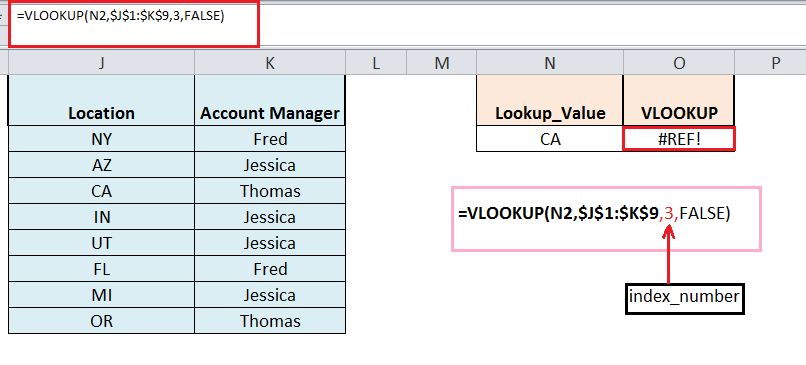### VLOOKUP returning incorrect results

If you omit to supply match type in a range_lookup argument of VLOOKUP then by default it searches for approximate match values, if it does not find exact match value. And if table_array is not sorted in ascending order by the first column, then VLOOKUP returns incorrect results.

Solution:

You must always supply a relevant match type in a range_lookup argument of VLOOKUP as TRUE or FALSE.  And in case of approximate match type (TRUE), you must always sort your table_array in ascending order by the first column of your table_array.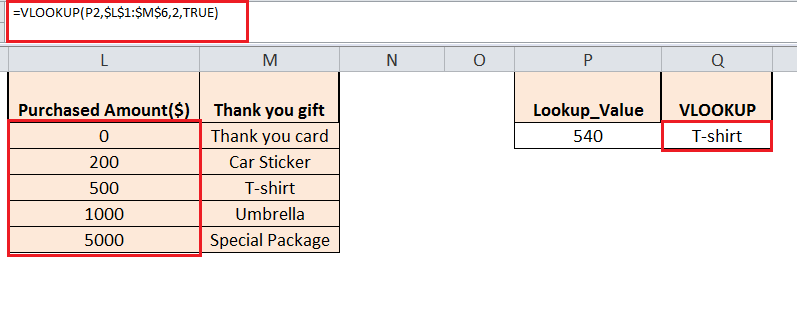Still need some help with Excel formatting or have other questions about Excel? Connect with a live Excel expert here for some 1 on 1 help. Your first session is always free.

Are you still looking for help with the VLOOKUP function? View our comprehensive round-up of VLOOKUP function tutorials here.

### Did this post not answer your question? Get a solution from connecting with the expert.Another blog reader asked this question today on Excelchat:
Solution examplesI need a formula to compare the data in two columns and then export the mismatched data in the 3rd column
Solved by S. Q. in 20 minsMy VLOOKUP formula won't work when value the "lookup cell" is derived from a formula. If I manually transfer the formula derived value to a different cell, then VLOOKUP formula works fine. What's going on?
Solved by Z. J. in 40 minsI need a formula that will compare multiple columns, and return the column header name of whichever column had the highest value. I am using (INDEX(\$F\$1:\$K\$1,0,MATCH(MAX(F2:K2),F2:K2,0))) , but one row had all the same values and the formula returned the first column header as being the "winner". This formula cannot distinguish when there are ties.
Solved by M. C. in 51 minsI filtered data in column A (it is labeled ID #) of a data set. On my next worksheet, column A is also the ID #, but it is a different data set. I want to filter the ID #'s the same for the two sheets, but how do I do that being that I am working with two different sets of data?
Solved by V. H. in 22 minsI'm looking for a formula or format for if there is data in c4 on a sheet... It adds extra information underneath
Solved by X. E. in 20 mins## Subscribe to Excelchat.coAnother blog reader asked this question today on Excelchat: